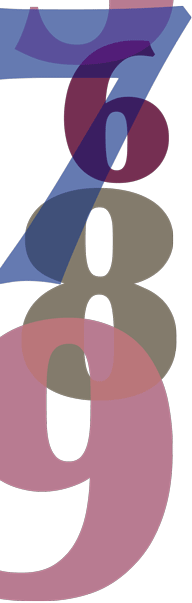# Year 6

## Number and Algebra

### Decimals

The word decimal comes from the Latin word decem meaning 'ten'. Decimal notation is a useful way of writing fractions with a denominator of ten, one hundred, one thousand and so on. For example, $$\dfrac{7}{10}$$ is written as 0.7, 8 $$\dfrac{3}{100}$$ is written as 8.03 and $$\dfrac{395}{1000}$$ is written as 0.395.

We use a base-10 number system. When we write numbers, each place tells us about the value of the digit. For example:

$$12.3456 = 1 \times 10 + 2 \times 1 + 3 \times \dfrac{1}{10} + 4 \times \dfrac{1}{100} + 5 \times \dfrac{1}{1000} + 6 \times \dfrac{1}{10000}$$

We can write 12.3456 in a place value chart to show the value of each place.

Place value chart
Tens Ones tenths hundredths thousandths tens of thousandths
1 2. 3 4 5 6

We use a decimal separator, known as a decimal point, to distinguish between the whole number and fractional parts. To the left of the decimal point are the whole number parts: ones, tens, hundreds, thousands and so on. To the right of the decimal point are the fractional parts: tenths, hundredths, thousandths and so on.

We use decimals every day in the world around us. Our money is based on a decimal system. We also use decimals in measurements such the height of the students in the class, the litres of petrol we put in our cars and the weight of parcels we send overseas. We can use decimals to express a number that requires a great deal of accuracy – the difference between the first and second place-getters in the 100-metre hurdles in the Olympic Games can be down to thousandths of a second!